Categories

Weights

Moving Items

#### Aggregations

Moodle allows you to aggregate your grades in different ways, depending on how you want your grades calculated.  There is a detailed list of aggregation strategies accompanied by the examples and the mathematics used to calculate grades.  Whether an instructor employs the Moodle grade book or not, having a clear understanding of exactly how a grade is calculated is essential to assigning grades.   Moodle allows for granular control of categories and weights in aggregating grades.  Moodle's documentation goes into detail.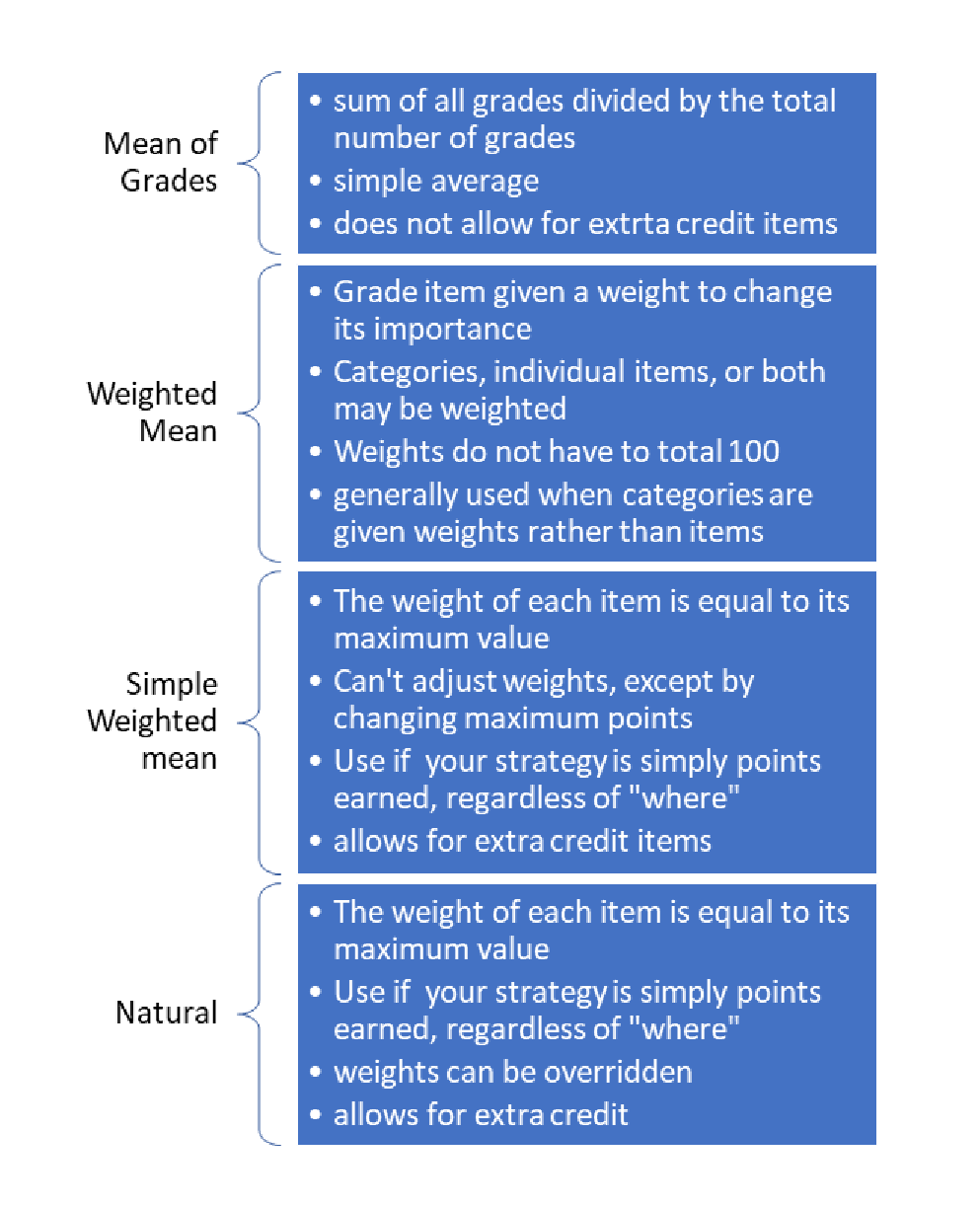### Example Calculations

 Raw Scores Normalized Score Assign 1 75/100 .75 Assign 2 35/50 .70 Assign 3 10/10 1 Assign 4 85/100 .85

All raw scores are first normalized to a value between 0 and 1:

####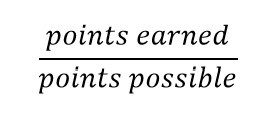Mean of Grades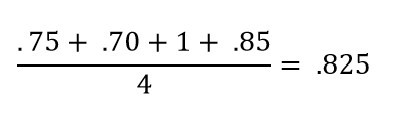#### Simple Weighted Mean

Normalized grade multiplied by weight, divided by sum of weights: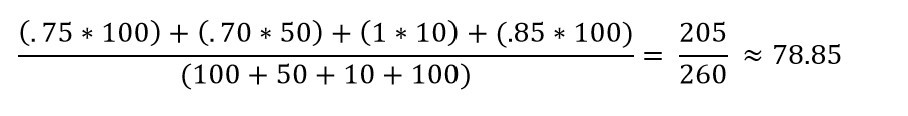This is equivalent to:#### Natural

If the weights are not adjusted, the default weight for each item is the maximum points possible, and the calculations are identical to Simple Weighted Mean. Natural aggregation allows for weights to be overridden.

#### Weighted Mean

 Category Percentage Weight Category Subtotal Daily Work 90% 30% .90 * 30 =  27 Participation 25% 10% .25 * 10 = 2.5 Tests 75% 50% .75 * 50 = 37.5 Final 65% 10% .65 * 10 = 6.5

First, Moodle calculates the percentage score in each category.  You may select whichever aggregation method you prefer for each category.  Categories may have different aggregation methods, which may be different from the overall aggregation method.

Final Calculation: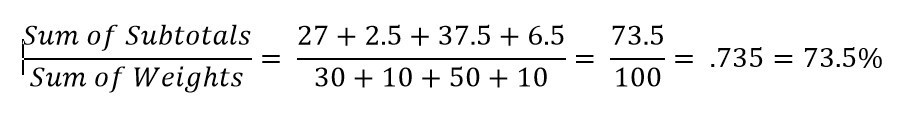The calculation may seem redundant because the weights add up to 100.  Moodle does not require that weights add up to 100 in this method:

 Category Percentage Weight Category Subtotal Daily Work 90% 1 .90 * 1 = .9 Participation 25% 1 .25 * 1 = .25 Tests 75% 3 .75 * 3 = 2.25 Final 65% 2 .65 * 10 = 1.3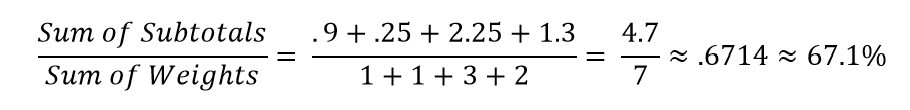(Note:  there was no attempt to use equivalent weights between the two examples)